# C173 – Wet Gas Venturi Calculation

## Description

This calculates the liquid and gas flow rate for a Venturi meter.

Kelton calculation reference C173

KIMS calculation reference K179

ISO 5167-1: Measurement of fluid flow by means of pressure differential devices inserted in circular cross-section conduits running full

Flow Measurement Engineering Handbook (2nd Edition) – R.W Miller

## Options

### Expansibility factor

• User Entered
• Calculated
• Liquid

The expansibility factor can either be user entered, a fixed value of 1 for a liquid or calculated.

### Correction

• None
• Murdock
• Chisholm
• User Entered

Select whether the Murdock, Chisholm or user entered correction is to be applied, if the Chisholm correction is applied you will be given the further option of calculating the De Leeuw ‘n’ term.

### Discharge Coefficient

• User Entered
• Variable with Reynold’s number
• Classical venturi with ‘as cast’ convergent section
• Classical venturi with machined convergent section
• Classical venturi with rough welded sheet iron convergent section
• Venturi Nozzle

Choose to either enter a fixed value for the discharge coefficient, one which varies with the Reynolds number or use the value which corresponds to the type of Venturi as referred to in the ISO 5167 standard.

• User entered
• Calculated

### Methanol Densit

• User entered
• Calculated

Note that if there is no methanol present select user entered and enter a non zero term for methanol density otherwise a divide by zero error will occur.

### Condensate Density

• User entered
• Calculated

If calculated is selected further options relating to density referral will appear to define how the condensate density is solved.

### Standard

• 1991
• 2003

This option allows selection of the relevant version of the ISO 5167 standard to be employed within the calculation.

### Exit Cone Angle

• 15°

This option is used to select exit cone angle. This uses the calculations set out by in the referenced Handbook by Miller.

### Temperature

• Upstream
• Downstream

This option is used to select whether the temperature entered is upstream or downstream of the venturi. If it downstream a correction, dependent on the standard version, will have to be made.

### Joule-Thomson

• User entered
• Calculated

If temperature is to be entered downstream and the ISO 5167:2003 is to be used then this option will prompt whether Joule-Thomson coefficient is to be entered by the user or calculated.

## Calculations

### Expansibility factor

The expansibility factor is calculated using the equation: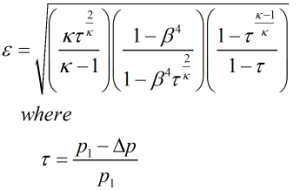Where κ = Isentropic exponent p1 = Line pressure Δp = Differential pressure β = Diameter ratio

### Mass Flow Rate

The general mass flow rate formula from ISO 5167 is used: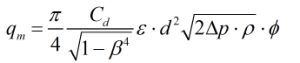Where Cd = Discharge coefficient β = Diameter ratio ε = Expansibility factor d = Throat diameter ΔP = Differential pressure ρ = Density of the fluid ϕ = Wet gas correction factor

### Chisholm correction

Chisholm correction is calculated from: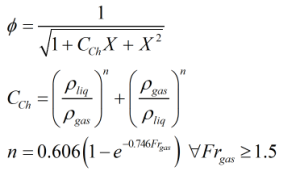Where ρliq = Density of the liquid ρgas = Density of the gas Frgas = Froude number of the gas X = Lockhart-Martinelli parameter n = Exponent of Chisholm coefficient, CCh, (n = 0.41 when Frgas < 1.5)

### Murdock Correction

The Murdock correction is calculated from: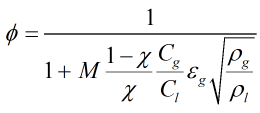Where M = Murdock coefficient Cgas = Gas discharge coefficient Cliq = Liquid discharge coefficient ε = Expansibility factor ρliq = Density of the liquid ρgas = Density of the gas mliq = Mass of the liquid mgas = Mass of the gas

### Lockhart-Martinelli Parameter

If ‘include expansibility term’ is selected then the Lockhart-Martinelli parameter is calculated from: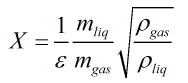Otherwise the Lockhart-Martinelli parameter is calculated from: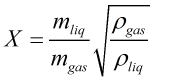Where ρliq = Density of the liquid ρgas = Density of the gas mliq = Mass of the liquid mgas = Mass of the gas

### Permanent Pressure Loss

The permanent pressure loss equations used here have been taken from R.W Miller’s Flow Measurement Engineering Handbook

For a venturi with an exit cone angle of 7°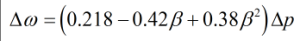For a venturi with an exit cone angle of 15°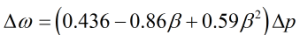### Correction to Upstream Temperature

If the 1991 standard is selected then upstream temperature is calculated by: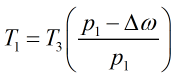If the 2003 standard is applied: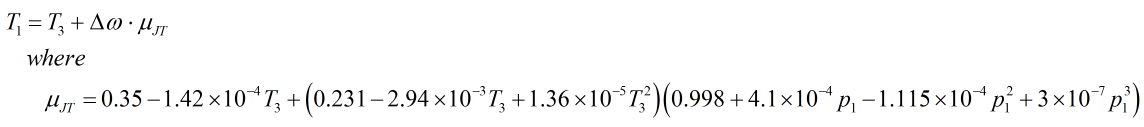Where p1 = Upstream pressure κ = Isentropic exponent T3 = Recovery point temperature Δω = Permanent pressure loss μJT = Joule-Thomson coefficient

Back to FLOCALC Calculations